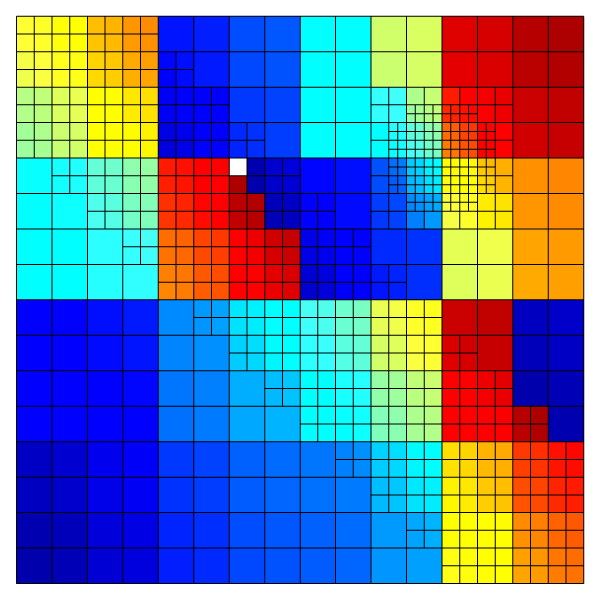# The tree-grid iterator

The page draws the cell-iterator movie

We use Bview to draw the process of grid-iteration

#include "view.h"

We visualize scalar field s as the iterator progresses.

int base_lev = 4, n, jj, nmin;
scalar s[];

Each iteration we will draw a frame with all the cells and the s field.

void draw_frame(char * fname){
cells();
squares ("s", min = 0, max = n);
save (fname);
}

int main(){

We define a camera-centered grid with some refinement features

  X0 = Y0 = -L0/2;
init_grid (1 << base_lev);
refine (fabs(x + y) < 0.2 && level <= base_lev);
refine (sq(x - 0.25) + sq(y - 0.25) < sq(0.1) && level < base_lev + 2);

We unset the data stored in s and count the number of cells for each thread.

  foreach(){
s[] = nodata;
n++;
}

For _MPI, each thread should help draw the same number of frames. therefore, the maximum number of cells is communicated between the processor ranks.

#if _MPI
MPI_Allreduce (&n, &nmin, 1, MPI_INT, MPI_MIN, MPI_COMM_WORLD);
MPI_Allreduce (MPI_IN_PLACE, &n, 1, MPI_INT, MPI_MAX, MPI_COMM_WORLD);
printf("%d %d %d\n", pid(), n, nmin);
//balance(); //<- This is already in refine()
#endif

We loop over the cells and per iteration we increase the value of s.

  view (fov = 20);
draw_frame("s.mp4");
foreach(){
s[] = jj;
draw_frame("s.mp4");
if (jj++ == nmin - 1 && nmin != 0)
draw_frame("unbalanced.png");
}

and continue untill all threads are finished.

  if (jj < n){
draw_frame("s.mp4");
jj++;
}
}White cell(s) indicate an unbalance